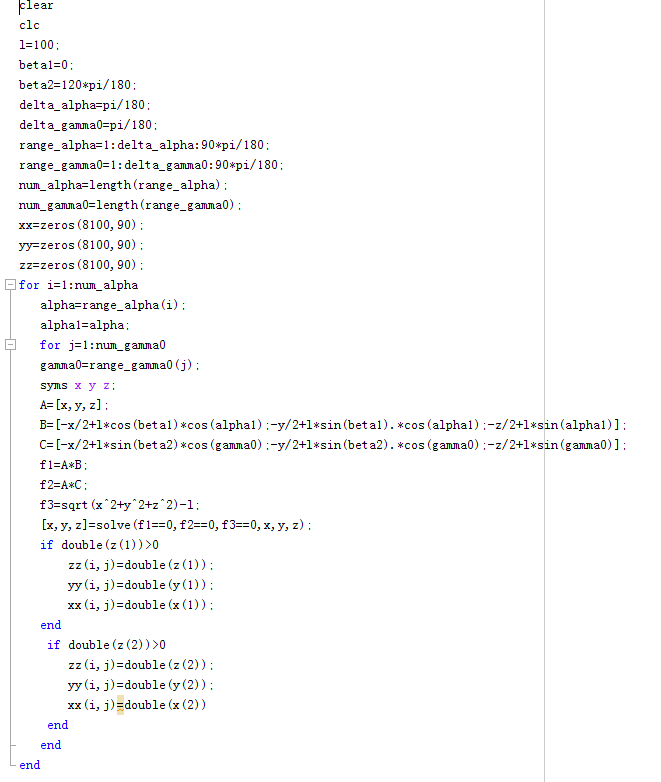matlab的for循环产生一个二维数组，如何把每一次的数据存到一个大数组里，以备后用？1个回答MATLAB里如何将存在二维数组/矩阵中的坐标点画出来

java调用matlab中调用二维数组问题

Matlab如何在二维数组中插入多行元素？

``` Array (  => Array ( [member_phone] => 13888888888 [ru_order_id] => Array ( ) [order_id] => Array ( ) [core_order_id] => Array (  => Array ( [order_id] => 20200310183831111111 ) ) )  => Array ( [member_phone] => 18888888888 [ru_order_id] => Array ( ) [order_id] => Array (  => Array ( [order_id] => 20200307145555555555 ) ) [core_order_id] => Array ( ) )  => Array ( [member_phone] => 17777777777 [ru_order_id] => Array ( ) [order_id] => Array ( ) [core_order_id] => Array ( ) )  => Array ( [member_phone] => 19999999999 ( ) [order_id] => Array ( ) [core_order_id] => Array (  => Array ( [order_id] => 202003022222222222 ) ) )  => Array ( [member_phone] => 12222222222 [ru_order_id] => Array ( ) [order_id] => Array ( ) [core_order_id] => Array ( ) )  => Array ( [member_phone] => 15555555555 [ru_order_id] => Array ( ) [order_id] => Array ( ) [core_order_id] => Array ( ) ) ） ``` 我现在想要如果ru_order_id，order_id，core_order_id这三个值都是空的 那么我就把这条记录拿掉。如果这三个任何一个有值 则保留 我应该怎么做才能得到这个数组

vc读取文件中的坐标后，把数据存储到一个四维的数组中，该怎么做？其中的x,y,z还要分别存储，该怎么区分呢？

matlab列数组在for循环中的一个小问题

exn是一个一维列数组 b=exn; a=114; for i=0:1:687: for j=1:149: a=a-1+j; b(a)=b(a)*(-1)^i; end end 最后报错 error in untitled b(a)=b(a)*(-1)^i; 请问下各位了解的，问题出在哪里呢

matlab三维数组合并 c=[a(1,:,:),a(2,:,:)]

c=[a(1,:,:),a(2,:,:)];像这样a(1,:,:)和a(2,:,:)会上下罗列变为一个数组，，但是我想变成左右排布，该怎么办:(

MATLAB中三维矩阵和二维矩阵如何拼接？

A是一个三维矩阵，其中每一页的矩阵是4*2的，B是2*2的二维矩阵，直接[A,B]MATLAB运行显示串联维度不同

matlab初级 数组删除问题求思路

<div class="post-text" itemprop="text"> <p>This is my 3 dimensional array.</p> <p>My \$_POST array. These are the values</p> <pre><code>\$_POST = Array (  =&gt; Array ( [student_id] =&gt; 9 [subject_id] =&gt; 6 [class_id] =&gt; 2 [mark_obtained] =&gt; 40 [mark_obtainable] =&gt; 100 )  =&gt; Array ( [student_id] =&gt; 10 [subject_id] =&gt; 6 [class_id] =&gt; 2 [mark_obtained] =&gt; 52 [mark_obtainable] =&gt; 100 )  =&gt; Array ( [student_id] =&gt; 11 [subject_id] =&gt; 6 [class_id] =&gt; 2 [mark_obtained] =&gt; 30 [mark_obtainable] =&gt; 100 )  =&gt; Array ( [student_id] =&gt; 12 [subject_id] =&gt; 6 [class_id] =&gt; 2 [mark_obtained] =&gt; 68 [mark_obtainable] =&gt; 100 ) ) </code></pre> <p>How do i loop through it and submit each of the array into the database?</p> <p>My column names are the keys. "<code>student_id</code>, <code>subject_id</code>, <code>class_id</code>, <code>mark_obtained</code>, <code>mark_obtainable</code>".</p> <p>I am trying this but i keep getting duplicate entries.`foreach (\$_POST as \$i =&gt; \$values1) {</p> <pre><code>foreach (\$values1 as \$key =&gt; \$value) { \$columns = implode(", ",array_keys(\$values1)); \$values = implode(", ", \$values1); \$columns = \$columns \$sql = "INSERT INTO `daily_report`(\$columns) VALUES (\$values)"; if (\$conn-&gt;multi_query(\$sql) === TRUE) { echo "New records created successfully &lt;br&gt;"; } else { echo "Error: " . \$sql . "&lt;br&gt;" . \$conn-&gt;error; } } </code></pre> </div>

2020阿里全球数学大赛：3万名高手、4道题、2天2夜未交卷

HashMap底层实现原理，红黑树，B+树，B树的结构原理 Spring的AOP和IOC是什么？它们常见的使用场景有哪些？Spring事务，事务的属性，传播行为，数据库隔离级别 Spring和SpringMVC，MyBatis以及SpringBoot的注解分别有哪些？SpringMVC的工作原理，SpringBoot框架的优点，MyBatis框架的优点 SpringCould组件有哪些，他们...

《经典算法案例》01-08：如何使用质数设计扫雷（Minesweeper）游戏

《Oracle Java SE编程自学与面试指南》最佳学习路线图（2020最新版）